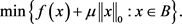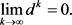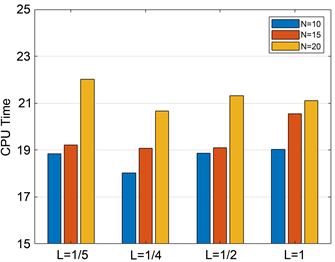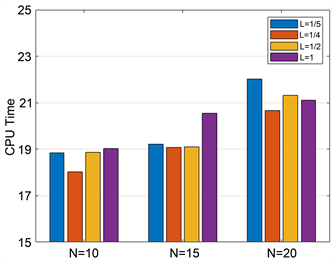﻿ 有效集方法求解欠定线性方程组的稀疏非负解

# 有效集方法求解欠定线性方程组的稀疏非负解Sparse Nonnegative Solution of Underdetermined Linear Equations by Active Set Method

Abstract: In this paper, for acquiring the sparse nonnegative solution of underdetermined linear equations, the original problem is relaxed into a l0 regularized optimization model. An active set identification technique is developed to accurately identify the zero components in a neighbourhood of the strict L-stationary point. Based on the active set identification technique, we propose an active set Barzilar-Borwein method to solve a l0 regularized minimization model. Numerical results show that the algorithm can effectively solve the sparse nonnegative solutions of underdetermined linear equations.

1. 引言

$\begin{array}{l}\mathrm{min}{‖x‖}_{0}\\ \text{s}\text{.t}\text{.}\text{\hspace{0.17em}}Ax=b,x\ge 0.\end{array}$ (1)

$\begin{array}{l}\mathrm{min}\varphi \left(x\right):=f\left(x\right)+\mu {‖x‖}_{0}\\ \text{s}\text{.t}\text{.}\text{\hspace{0.17em}}x\ge 0.\end{array}$ (2)

2. 符号说明

$\gamma \left({x}^{*}\right)=\left\{i:{x}_{i}^{*}=0\right\}和\tau \left({x}^{*}\right)=\left\{i:{x}_{i}^{*}>0\right\}.$

${h}_{0}{\left(\nu ,\alpha \right)}_{i}=\left\{\begin{array}{l}{\nu }_{i},|{\nu }_{i}|>\sqrt{2\alpha },\\ 0,|{\nu }_{i}|\le \sqrt{2\alpha }.\end{array}\text{\hspace{0.17em}}\text{\hspace{0.17em}}i=1,2,\cdots ,n.$

${h}_{0}\left(\nu ,\alpha \right)=\underset{u\in {R}^{n}}{\mathrm{arg}\mathrm{min}}\left\{{‖u‖}_{1}:u=\underset{x\in {R}^{n}}{\mathrm{arg}\mathrm{min}}\left\{\alpha {‖x‖}_{0}+\frac{1}{2}{‖x-\nu ‖}^{2}\right\}\right\}.$(3)

${g}_{i}\left({x}^{*}\right)=0,\forall i\in \tau \left({x}^{*}\right).$ (4)

$\begin{array}{l}{x}^{*}\in pro{x}_{\frac{\mu }{L}{g}_{B}}\left({x}^{*}-\frac{1}{L}g\left({x}^{*}\right)\right)\\ =\underset{x\in B}{\mathrm{arg}\mathrm{min}}\left\{\frac{\mu }{L}{‖x‖}_{0}+\frac{1}{2}{‖x-\left({x}^{*}-\frac{1}{L}g\left({x}^{*}\right)\right)‖}^{2}\right\}.\end{array}$

${\delta }_{B}\left(x\right)=\left\{\begin{array}{l}0,x\in B;\\ 1,x\notin B.\end{array}$

$B={R}_{+}^{n}$ 时，问题 等价于问题 ，则上述条件等价于下面的条件：

$\left\{\begin{array}{l}\text{0}\le {g}_{i}\left({x}^{*}\right)\le \sqrt{2\mu L},i\in \gamma \left({x}^{*}\right);\\ {x}_{i}^{*}\ge \sqrt{\frac{2\mu }{L}}且{g}_{i}\left({x}^{*}\right)=0,i\in \tau \left({x}^{*}\right).\end{array}$

$\left\{\begin{array}{l}\text{0}\le {g}_{i}\left({x}^{*}\right)\le \sqrt{2\mu L},i\in \gamma \left({x}^{*}\right);\\ {x}_{i}^{*}>\sqrt{\frac{2\mu }{L}}且{g}_{i}\left({x}^{*}\right)=0,i\in \tau \left({x}^{*}\right).\end{array}$ (5)

$\left\{\begin{array}{l}\text{0}\le {g}_{i}\left({x}^{*}\right)\le \sqrt{2\mu L},i\in \gamma \left({x}^{*}\right);\\ {g}_{i}\left({x}^{*}\right)=0,i\in \tau \left({x}^{*}\right).\end{array}$

$\varphi \left(x+\alpha d\right)<\varphi \left(x\right),\forall \alpha \in \left(0,\delta \right).$

$D\left(x\right)=\left\{d\in {R}^{n}|\varphi \left(x+\alpha d\right)<\varphi \left(x\right),\forall \alpha \in \left(0,\delta \right)\right\}.$

$D\left(x\right)=\left\{d\in {R}^{n}|{g}_{\tau \left(x\right)}^{\text{T}}{d}_{\tau \left(x\right)}<0,{d}_{\gamma \left(x\right)}=0\right\}.$

3. 有效集估计

$A\left(x\right)=\left\{i:0\le {x}_{i}\le \frac{1}{L}{g}_{i}\left(x\right)+\sqrt{\frac{2\mu }{L}}\right\},$

$F\left(x\right)=\left\{i:{x}_{i}>\frac{1}{L}{g}_{i}\left(x\right)+\sqrt{\frac{2\mu }{L}}\right\}.$

$A\left({x}^{k}\right)\subseteq \gamma \left({x}^{*}\right)且\tau \left({x}^{*}\right)\subseteq F\left({x}^{k}\right).$

$A\left({x}^{k}\right)\cup F\left({x}^{k}\right)=\gamma \left({x}^{*}\right)\cup \tau \left({x}^{*}\right)=\left\{1,2,\cdots ,n\right\}.$

${x}_{i}^{*}-\frac{1}{L}{g}_{i}\left({x}^{*}\right)={x}_{i}^{*}>\sqrt{\frac{2\mu }{L}}.$

${x}_{i}^{k}-\frac{1}{L}{g}_{i}\left({x}^{k}\right)>\sqrt{\frac{2\mu }{L}}.$

$0\le {x}_{i}^{k}\le \frac{1}{L}{g}_{i}\left({x}^{k}\right)+\sqrt{\frac{2\mu }{L}}.$

$0\le {x}_{i}^{*}\le \frac{1}{L}{g}_{i}\left({x}^{*}\right)+\sqrt{\frac{2\mu }{L}}.$

$A\left({x}^{k}\right)=\gamma \left({x}^{k}\right),F\left({x}^{k}\right)=\tau \left({x}^{k}\right).$

${x}_{i}^{k}\le \sqrt{\frac{2\mu }{L}}⇒{x}_{i}^{k}=0.$

4. 有效集算法

4.1. 搜索方向的一些性质

${x}^{k}\in \Omega$ 是第k个迭代点，为了简化表述，令

${A}^{k}=A\left({x}^{k}\right),{F}^{k}=\left\{i\in F\left({x}^{k}\right):{x}_{i}>0\right\}.$

${A}_{1}^{k}=\left\{i\in {A}^{k}:{x}_{i}^{k}{g}_{i}\left({x}^{k}\right)\ge 0\right\},{A}_{2}^{k}=\left\{i\in {A}^{k}:{x}_{i}^{k}{g}_{i}\left({x}^{k}\right)<0\right\}.$

${d}_{i}^{k}=-{\lambda }^{k}{g}_{i}^{k},i\in {F}^{k}.$ (6)

${d}_{i}^{k}=-{x}_{i}^{k},i\in {A}_{1}^{k}.$ (7)

${d}_{i}^{k}=-{g}_{i}^{k},i\in {A}_{2}^{k}.$ (8)

${x}_{i}^{k}=0且{g}_{i}\left({x}^{k}\right)\le \sqrt{2\mu L}.$

${x}_{i}^{k}>\sqrt{\frac{2\mu }{L}}且{g}_{i}\left({x}^{k}\right)=0.$

${g}_{i}\left({x}^{k}\right)=0且{x}_{i}^{k}\le \sqrt{\frac{2\mu }{L}}⇒{x}_{i}^{k}=0.$

${g}_{i}\left({x}^{k}\right)=0且{d}_{i}^{k}=0.$

${x}_{i}^{*}=0且{g}_{i}\left({x}^{*}\right)\le \sqrt{2\mu L},$

${g}_{i}\left({x}^{*}\right)=0.$

${x}_{i}^{*}\ge \sqrt{\frac{2\mu }{L}}且{g}_{i}\left({x}^{*}\right)=0.$

${\left({g}^{k}\right)}^{\text{T}}{d}^{k}\le 0.$ (9)

${g}_{i}^{k}{d}_{i}^{k}=-{g}_{i}^{k}{x}_{i}^{k}\le 0,\forall i\in {A}_{i}^{k}.$

${g}_{i}^{k}{d}_{i}^{k}=-{\left({g}_{i}^{k}\right)}^{2}<0.$

${\left({g}_{i}^{k}\right)}^{\text{T}}{d}_{i}^{k}\le -{\lambda }_{\mathrm{min}}{‖{\left({g}_{i}^{k}\right)}^{\text{T}}‖}^{2}\le 0.$

${g}_{i}\left({x}^{k}\right)=0且{x}_{i}^{k}>\sqrt{\frac{2\mu }{L}},\forall i\in {F}^{k}.$

${A}_{2}^{k}$ 的定义可知 ${A}_{2}^{k}=\varnothing$。由的定义可得：

${x}_{i}^{k}=0,0\le {g}_{i}^{k}\le \sqrt{2\mu L}或0<{x}_{i}^{k}\le \sqrt{\frac{2\mu }{L}},{g}_{i}^{k}=0.$

4.2. 算法

$\varphi \left({x}^{k}+{\alpha }^{k}{d}^{k}\right)\le \varphi \left({x}^{k}\right)-\delta {\left({\alpha }^{k}‖{d}^{k}‖\right)}^{2}.$ (10)

$f\left({x}^{k}+{\alpha }^{k}{d}^{k}\right)\le \underset{0\le j\le m\left(k\right)}{\mathrm{max}}f\left({x}^{k-j}\right)-\delta {\left({\alpha }^{k}‖{d}^{k}‖\right)}^{2}.$ (11)

$\underset{\lambda \in R}{\mathrm{min}}{‖D\left(\lambda \right){s}_{{F}^{k}}^{k-1}-{y}_{{F}^{k}}^{k-1}‖}^{2}.$

${\lambda }_{BB}^{k}=\frac{{‖{s}_{{F}^{k}}^{k-1}‖}^{2}}{{\left({s}_{{F}^{k}}^{k-1}\right)}^{\text{T}}{y}_{{F}^{k}}^{k-1}}.$

${\lambda }^{k}=\mathrm{max}\left\{{\lambda }_{\mathrm{min}},\mathrm{min}\left\{{\lambda }_{\mathrm{max}},{\lambda }_{BB}^{k}\right\}\right\}$ (12)

4.3. 全局收敛性分析

$\underset{k\to \infty }{\mathrm{lim}}{\alpha }^{k}{d}^{k}=0.$ (13)

$\underset{k\to \infty }{\mathrm{lim}}{x}^{k}={x}^{*}.$$\underset{k\to \infty }{\mathrm{lim}}{d}^{k}=0.$

$f\left({x}^{k}+\frac{{\alpha }^{k}}{\eta }{d}^{k}\right)-f\left({x}^{k}\right)\ge f\left({x}^{k}+\frac{{\alpha }^{k}}{\eta }{d}^{k}\right)-\underset{0\le j\le m\left(k\right)}{\mathrm{max}}f\left({x}^{k-j}\right)>-\delta {\left(\frac{{\alpha }^{k}}{\eta }‖{d}^{k}‖\right)}^{2}.$ (14)

$f\left({x}^{k}+\frac{{\alpha }^{k}}{\eta }{d}^{k}\right)-f\left({x}^{k}\right)=\frac{{\alpha }^{k}}{\eta }{\left(g\left({x}^{k}+{\theta }^{k}\frac{{\alpha }^{k}}{\eta }{d}^{k}\right)-g\left({x}^{k}\right)\right)}^{\text{T}}{d}^{k}+\frac{{\alpha }^{k}}{\eta }g{\left({x}^{k}\right)}^{\text{T}}{d}^{k}.$

${\left(g\left({x}^{k}+{\theta }^{k}\frac{{\alpha }^{k}}{\eta }{d}^{k}\right)-g\left({x}^{k}\right)\right)}^{\text{T}}{d}^{k}+g{\left({x}^{k}\right)}^{\text{T}}{d}^{k}>-\delta \frac{{\alpha }^{k}}{\eta }{‖{d}^{k}‖}^{2}.$

5. 数值实验

${x}_{{\mu }_{k}}^{*}:=\underset{x\in {R}_{+}^{n}}{\mathrm{arg}\mathrm{min}}\left\{{\varphi }_{{\mu }_{k}}\left(x\right)=f\left(x\right)+{\mu }_{k}{‖x‖}_{0}\right\}.$

${\lambda }^{0}=1,M=10,{\lambda }_{\mathrm{min}}={10}^{-3},{\lambda }_{\mathrm{max}}=10,\delta ={10}^{-2},\eta =0.5$。首先用标准高斯分布的独立样本填充 $m×n$ 的度量矩阵A，并且对这些行进行正交化。而真实解 ${x}^{*}$ 是一个具有有效集 ${A}^{*}$ 的T-稀疏信号，观测向量b定义为：

$b=A{x}^{*}+e$Table 1. n = 5 × 10 3 , L = 1 / 5 , the results of ABB algorithm to solve the problem

Table 2. n = 5 × 10 3 , L = 1 / 4 , the results of ABB algorithm to solve the problemTable 3. n = 5 × 10 3 , L = 1 / 2 , the results of ABB algorithm to solve the problemTable 4. n = 5 × 10 3 , L = 1 , the results of ABB algorithm to solve the problemFigure 1. The influence of the change of N value on the average number of iterations at different L levelsFigure 2. The influence of the change of N value on operation time at different L levelsFigure 3. The influence of the change of L value on the average number of iterations at different N levelsFigure 4. The influence of the change of L value on operation time at different N levelsTable 5. n = 10 4 , L = 1 / 5 , the results of ABB algorithm to solve the problemTable 6. n = 10 4 , L = 1 / 4 , the results of ABB algorithm to solve the problemTable 7. n = 10 4 , L = 1 / 2 , the results of ABB algorithm to solve the problemTable 8. n = 10 4 , L = 1 , the results of ABB algorithm to solve the problem

6. 小结

NOTES

*第一作者。

#通讯作者。

 Donoho, D.L. (2006) Compressed Sensing. IEEE Transactions on Information Theory, 52, 1289-1306.
https://doi.org/10.1109/TIT.2006.871582

 Bruckstein, A.M., Donoho, D.L. and Elad, M. (2009) From Sparse Solutions of Systems of Equations to Sparse Modeling of Signals and Images. SIAM Review, 51, 34-81.
https://doi.org/10.1137/060657704

 Candès, E. and Tao, T. (2006) Near Optimal Signal Recovery from Random Projections: Universal Encoding Strategies. IEEE Transactions on Information Theory, 52, 5406-5425.
https://doi.org/10.1109/TIT.2006.885507

 Tropp, J.A. and Gilbert, A. (2007) Signal Recovery from Random Measurements via Orthogonal Matching Pursuit. IEEE Transactions on Information Theory, 53, 4655-4666.
https://doi.org/10.1109/TIT.2007.909108

 Needell, D. and Vershynim, R. (2010) Signal Recovery from In-complete and Inaccurate Measurements via Regularized Orthogonal Matching Pursuit. IEEE Journal of Selected Topics in Signal Processing, 4, 310-316.
https://doi.org/10.1109/JSTSP.2010.2042412

 Needell, D. and Tropp, J.A. (2008) CoSaMP: Iterative Signal Recovery from Incomplete and Inaccurate Samples. Applied and Computational Harmonic Analysis, 26, 301-321.
https://doi.org/10.1016/j.acha.2008.07.002

 Dai, W. and Milenkovic, O. (2009) Subspace Pursuit for Com-pressive Sensing Signal Reconstruction. IEEE Transactions on Information Theory, 55, 2230-2249.
https://doi.org/10.1109/TIT.2009.2016006

 Candès, E., Wakin, M.B. and Boyd, S.P. (2008) Enhancing Spar-sity by Reweighted L1 Minimization. Journal of Fourier Analysis and Applications, 14, 877-905.
https://doi.org/10.1007/s00041-008-9045-x

 Gorodnitsky, I.F. and Rao, B.D. (1997) Sparse Signal Recon-struction from Limited Data Using FOCUSS: A Reweighted Minimum Norm Algorithm. IEEE Transactions on Signal Processing, 45, 600-616.
https://doi.org/10.1109/78.558475

 Cheng, W., Chen, Z. and Hu, Q. (2019) An Active Set Barzilar-Borwein Algorithm for L0 Regularized Optimization. Journal of Global Optimization, 76, 769-791.
https://doi.org/10.1007/s10898-019-00830-w

 Zeng, J.S., Lin, S.B. and Xu, Z.B. (2014) Sparse Solution of Underdetermined Linear Equations via Adaptively Iterative Thresholding. Signal Processing, 97, 152-161.
https://doi.org/10.1016/j.sigpro.2013.10.031

 Beck, A. and Hallak, N. (2018) Proximal Mapping for Sym-metric Penalty and Sparsity. SIAM Journal on Optimization, 28, 496-527.
https://doi.org/10.1137/17M1116544

 Grippo, L., Lampariello, F. and Lucidi, S. (1986) A Non-Monotone Line Search Technique for Newton’s Method. SIAM Journal of Numerical Analysis, 23, 707-716.
https://doi.org/10.1137/0723046

 Barzilai, J. and Borwein, J.M. (1988) Two Point Step Size Gradient Method. IMA Journal of Numerical Analysis, 8, 141-148.
https://doi.org/10.1093/imanum/8.1.141

 Jiao, Y.L., Jin, B.J. and Lu, X.L. (2015) A Primal Dual Active Set with Continuation Algorithm for the L0 Regularized Optimization Problem. Applied and Computational Harmonic Analysis, 39, 400-426.
https://doi.org/10.1016/j.acha.2014.10.001

Top